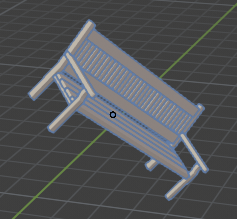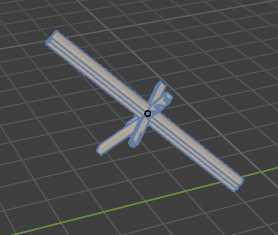# Importing, Setting Pivot Point, Locating and Rotating

Hello,

I’m trying to import a model, set its location it and rotate the whole model using the quaternion mode.

Here’ s my code:

``````bpy.ops.object.select_all(action='SELECT')
bpy.ops.object.delete()
bpy.ops.import_scene.obj(filepath='Bench_1.obj', axis_forward='-Z', axis_up='Y')
object = bpy.context.selected_objects
for obj in bpy.context.selected_objects:
if obj.type == 'MESH':
obj.location.x = 5
obj.location.z = 5
obj.location.y = 5
obj.rotation_mode = "QUATERNION"
obj.rotation_quaternion.x = 1
obj.rotation_quaternion.y = 1
obj.rotation_quaternion.z = 0
obj.rotation_quaternion.w = 1
bpy.context.scene.update()
``````

It works fine, but in this case model’s pivot point is right in the center.Some other models may have pivot point far away from geometry, so I can’t neither locate, nor rotate them properly. I decided to put an extra line in my code that will always set pivot point in the center of any imported model:

``````bpy.ops.object.select_all(action='SELECT')
bpy.ops.object.delete()
bpy.ops.import_scene.obj(filepath='Bench_1.obj', axis_forward='-Z', axis_up='Y')
object = bpy.context.selected_objects
bpy.ops.object.origin_set(type='ORIGIN_CENTER_OF_MASS') # EXTRA LINE HERE!
for obj in bpy.context.selected_objects:
if obj.type == 'MESH':
obj.location.x = 5
obj.location.z = 5
obj.location.y = 5
obj.rotation_mode = "QUATERNION"
obj.rotation_quaternion.x = 1
obj.rotation_quaternion.y = 1
obj.rotation_quaternion.z = 0
obj.rotation_quaternion.w = 1
bpy.context.scene.update()
``````

After this update my ‘normal’ models become fractured:What am I doing wrong?

P.S. Source model:
Bench.zip (13.4 KB)